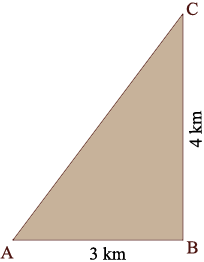SEARCH HOMEMath Central Quandaries & QueriesQuestion from Kayode A boy walks 3km due east and 4km due north. Find the bearing of the final point and the distance of the final position from the starting point.Hi Kayode,

I drew a diagram of the situation you described.Pythagoras Theorem will tell you the distance from the starting point A to to final position C.

For the bearing you need the measure of the angle CAB. What trig function relates the measure of the angle CAB to the lengths of the sides BC and AB? Use this trig function to determine the measure of the angle CAB.

HarleyMath Central is supported by the University of Regina and The Pacific Institute for the Mathematical Sciences.